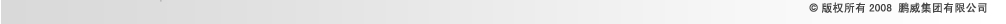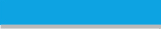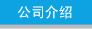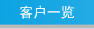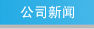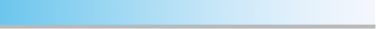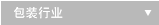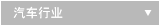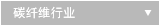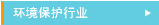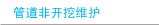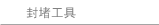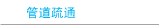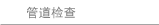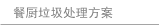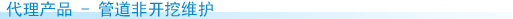管道疏通 - 水处理组合式管道清理车

 经济型车载水处理系统： 由双轴、三轴或四轴的载重货车和不同容积的储存罐组 成，其规格包括：容积10000l、额定载重18t；容积 14500l、额定载重26t；容积20000l、额定载重32t 专业型车载水处理系统： 由双轴、三轴或四轴的载重货车和不同容积的储存罐组成，其规格包括：容积12000l（7000+5000）、额定载 重18t；容积16000l（10000+6000）、额定载重26t； 容积18000l（12000+6000）、额定载重32t

• 住宅和市政管道的清理
• 大截面管道的清理
• 填埋和虹吸管的清理
• 特殊用途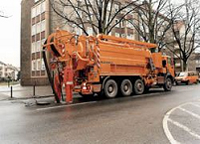• 带有过滤装置的水处理系统
• 圆柱形多槽储存罐
• 储存罐材料：碳钢或不锈钢
• 可作为隔离墙的排放活塞（3级）
• 可配置多种类型的真空泵： 回轮式真空泵、水环式真空泵 （单泵和双泵系统）
• 可配置各种类型泵： 三活塞泵、高压泵（单泵系统、双泵系统）
• 可配备降噪音装置
• 多种驱动方式：皮带驱动、液压驱动
• 多种储存罐排空方式：压力排空储存罐、重力排空储存罐、强制排空
• 多种储存罐阀门：手轮阀门； 液压楔形阀门
• 可配备多种管型（管长、管径和截面）
• 可配备多种收管架
• 暖气系统，用于冬季作业
• 高压泵的压力、转速控制
• 针对该类清理车，米勒公司提供采用标准元件的各种配置，可根据具体清理任务选择。

• 有效提高单位时间处理能力，可保证持续工作
• 节约成本，保护饮用水资源
• 通过过滤作用提高污泥槽的充满度
• 结构简单，维护方便
• 过滤槽通过旋转装置控制操作简单效果好
• 专业型：沉淀效果更好，水质更好，回用率更高
• 专业型：载重货车轴压力分配更理想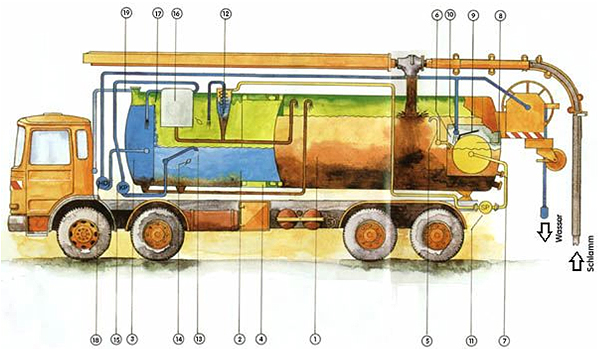1= 污泥槽 2= 沉淀槽 3= 水槽 4= 排放活塞 5= 带有浮漂的摆动管 6= 过滤槽 7= 过滤槽导管 8= 旋转过滤装置 9= 滤网清理装置 10= 滤网冲洗装置 11= 活塞泵 12= 细过滤 13= 带有浮漂的摆动管 14= 浮漂控制开关 15= 螺旋泵 16= 选择附加的精细过滤 17= 溢流 18= 高压泵 19= 备用管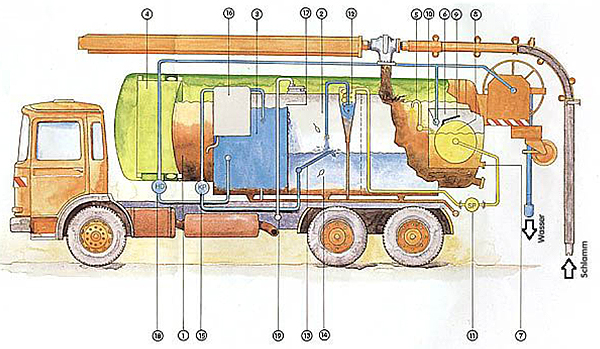1= 污泥槽 2= 沉淀槽 3= 水槽 4= 排放活塞 5= 带有浮漂的摆动管 6= 过滤槽 7= 过滤槽导管 8= 旋转过滤装置 9= 滤网清理装置 10= 滤网冲洗装置 11= 活塞泵 12= 细过滤 13= 带有浮漂的摆动管 14= 浮漂控制开关 15= 螺旋泵 16= 选择附加的精细过滤 17= 溢流 18= 高压泵 19= 备用管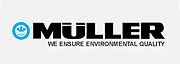真空清理车
危险类液态垃圾清理车
高压水清理车
一体化清理车
组合式管道清理车
水处理组合式管道清理车
工业清理车
各类附件